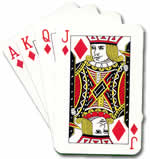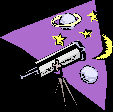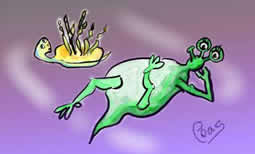# Card Shuffle

##### Age 11 to 16

Published 2006 Revised 2009.

Today I made a calculation which really surprised me.
It concerns the number of ways a pack of cards can be shuffled...

The number of ways is equal to:

$52!$, or approximately $8.07 \times 10^{67}$

$52!$, or $52$ factorial, means $52 \times51 \times50 \times49 \times\ldots \times3 \times2 \times1$

To work this out, I used the factorial button on my calculator and rounded the answer to 3 significant figures. You could also use Stirling's approximation (see the Notes).

The exact figure would certainly have a number of noughts at the end but certainly not 67 of them. You might like to work out just how many zeros there actually are at the end of the number 52!
But just how big is this number?

I wanted to get an idea of what this might be like and decided that a good way to do that might be to consider how long it would take to recreate every possible shuffle. I had this feeling that I might need some help so thought about how many people I might need to help me get it done in a reasonable time span.

To make things easy, let us assume that a person would be able to recreate one new shuffle every second.

There are 60 seconds in a minute, 60 minutes in an hour and 24 hours in a day. So...

In one day one person could generate:

$60 \times 60 \times24 = 86400 = 8.64 \times10^4$ shuffles.

There are 365 days in a year, so if I worked non stop I could manage:

$365 \times86400 = 31536000 = 3.15 \times10 ^7$ shuffles in one year.

Still feels like a long way to go! I need a lot more time so let's think about the age of the universe, that might help if I could live that long!

The universe is estimated to be between $12$ and $20$ billion years old. Let's take its age, for the sake of this calculation, to be $15$ billion years old. That is $1.5 \times 10^{10}$ years.

This means that, if I started doing the shuffles when the universe began, I would still be doing them now and I would have done:

$3.15 \times 10^7 \times 1.5 \times 10^{10} = 4.73 \times 10^{17}$ shuffles.

I think I will need some help!Our galaxy contains approximately $100,000,000,000 = 1.00 \times 10^{11}$ stars (suns).

There are approximately $100,000,000,000= 1.00 \times 10^{11}$ galaxies in the universe.

So there are approximately $1.00 \times10^{22}$ stars in our universe.

This is looking promising! But I think we still have some way to go.

Let's assume that around each star there circles one earth-like planet (that is a planet with alien life on it). This would mean that the number of Earths in the universe is $10^{22}$.

There are approximately $7$ billion people living on the Earth ($7.00 \times10^9$).

So there could be $7.00 \times10^9 \times1.00 \times10^{22} = 7.00 \times10^{31}$ aliens living in the universe.
So, I could utilise the population of the universe to help me!

Let's imagine that all these aliens have been living since the big bang and have been shuffling away. All they have been doing since the beginning of time is shuffling their pack of cards - one permutation per second.

The number of permutations tried by these aliens is therefore:

$7.00 \times10^{31} \times4.73 \times10^17 =3.31 \times10^{49}$

Which is only a fraction of all the possible permutations of a single pack of cards.

This is possibly why we have not been contacted by aliens yet - they are all too busy shuffling packs of cards!